top of page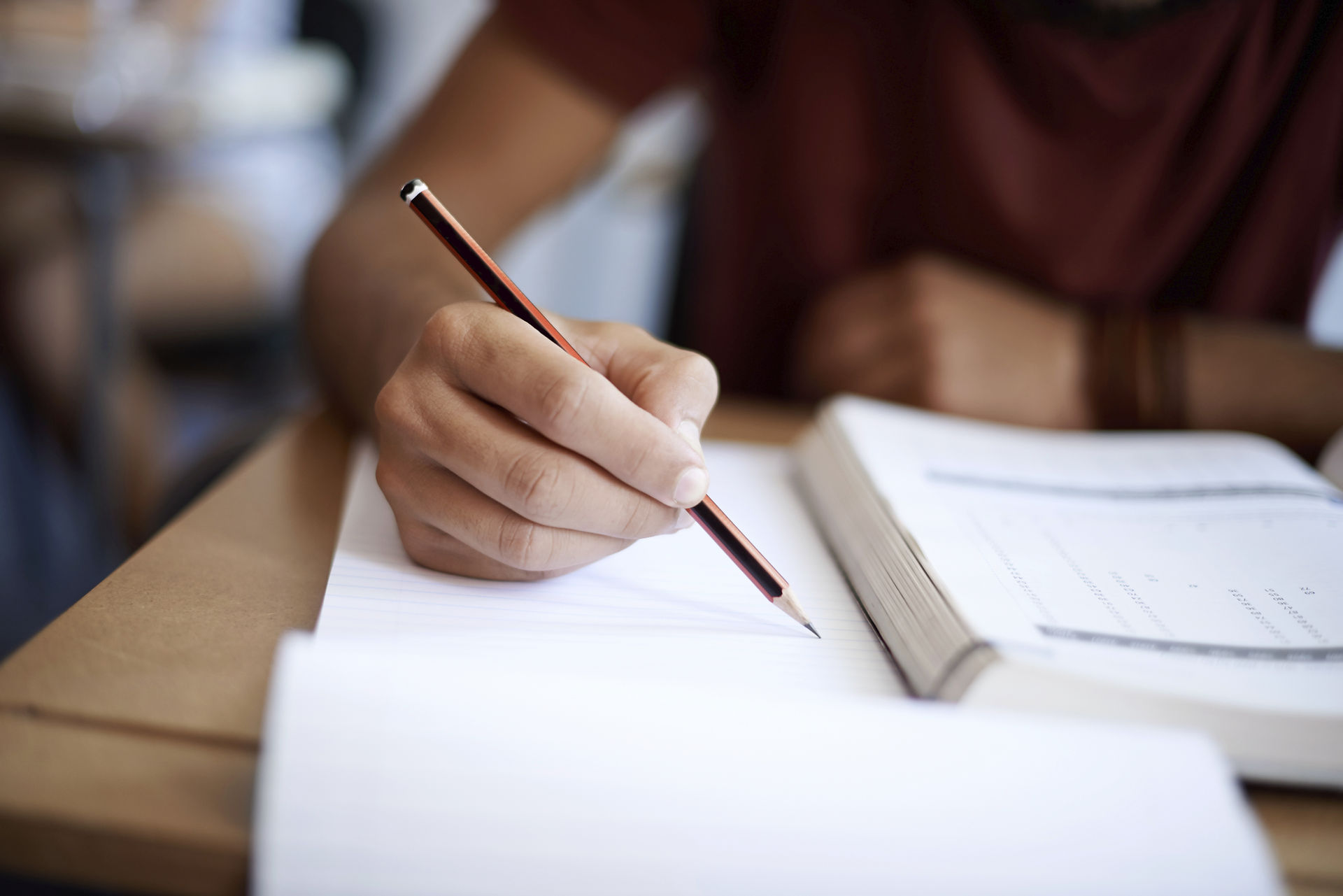# Av = λv           ................ (i)

Eigenvectors and eigenvalues# For example if A and v are as follows: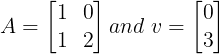8. # For a column where element aii ≠ 0 and all other elements aij = 0, one of the eigenvector is that column vector and corresponding eigenvalue is equal to magnitude of the column vector. For example for a 3x3 matrix as given below:# D) 3

GATE previous year questiond# |P - λI| = 0# GATE 2019 (1 Mark): In matrix equation [A]{X} = {R}, one of the eigenvalues of matrix A is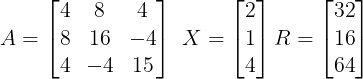# GATE 2017 (1 Mark): Product of eigenvalues of matrix P is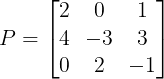# GATE 2017 (2 Marks): Consider the matrix A whose eigenvectors corresponding to eigenvalues λ1 and λ2 are x1 and x2 respectively. The value of (transpose of x1)*(x2) is _________.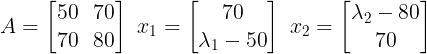# Solution:# GATE 2016 (2 Marks): The number of linearly independent eigenvectors of matrix A is ________.# GATE 2016 (1 Marks): The condition for which eigenvalues of the matrix A are positive, is# GATE 2014 (1 Mark):Consider a 3 × 3 real symmetric matrix S such that two of its eigen values are a ≠ 0 , b ≠ 0 with respective eigenvectors X and Y. If a ≠ b then x1y1 + x2y2 + x3y3 equals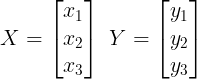# GATE 2012 (2 Marks): For the matrix A, one of the normalized eigenvector is given as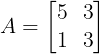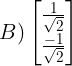# (A - 2I)X = 0# Eigenvector corresponding to λ = 2 is# Eigenvector in normalized form is# GATE 2008 (2 Marks): The eigenvectors of the matrix A as given below are written in the form# (A - 2I)X = 0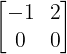# So two eigenvectors may be written as# GATE 2006 (2 Marks): Eigenvalue of a matrix S are 5 & 1. What are the eigenvalues of matrix S*S.# GATE 2005 (2 Marks): Which one of the following is an eigenvector of the matrix.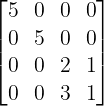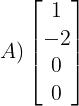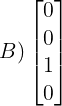# For option A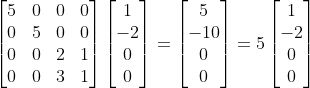bottom of page